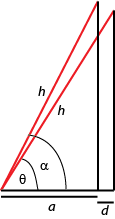# Trig Reps

##### Age 16 to 18Challenge Level

The trigonometric functions $\sin(x)$ and $\cos(x)$ are of fundamental importance in advanced mathematics, both pure and applied. They have many beautiful mathematical properties. Sine and cosine are usually first encountered in the context of right-angled triangles, but mathematicians usually use alternative definitions for more advanced work.

Here are three different ways of representing the sine and cosine functions

1. Through ratios of the sides of right angled triangles:$\cos(\theta) = \frac{a+d}{h}\quad \cos(\alpha) = \frac{a}{h}$

$\sin(\theta) = \frac{\sqrt{h^2-(a+d)^2}}{h}\quad\quad\sin(\alpha) = \frac{\sqrt{h^2-a^2}}{h}$

2. Through power series:

$$\cos(x) = 1-\frac{x^2}{2!}+\frac{x^4}{4!}-\frac{x^6}{6!}+\cdots\quad\quad \sin(x) = x-\frac{x^3}{3!}+\frac{x^5}{5!}-\frac{x^7}{7!}+\cdots$$

3. Through $e$ and $i$:

$$\cos(x) = \frac{e^{i x} + e^{-ix}}{2}\quad\quad \sin(x) = \frac{e^{ix}-e^{-ix}}{2i}$$

How do these different definitions give rise to the familiar properties of trigonometry functions? Some representations give some of the properties very easily, whereas you might find it very difficult or even impossible to deduce some of the properties with one or more of the representations!

Tick off the cells in the table when you manage to find proofs noting whether the representation made it easy or difficult to deduce that property.

 Property Triangles Power Series $e$ and $i$ $(\cos x)^2+(\sin x)^2 =1$ $\cos(0) =1 ,\quad \sin(0)=0$ $\sin(a+b)=\sin(a)\cos(b)+\cos(a)\sin(b)$ $\cos(2x) = 2\cos^2(x)-1$ $\cos(x+\pi) = -\cos(x),\quad \sin(x+\pi) = -\sin(x)$ $\frac{d}{dx}(\sin(x)) = \cos(x), \quad \frac{d}{dx}(\cos(x)) = -\sin(x)$ $\cos\left(\frac{\pi}{2}\right) = 0, \quad \sin\left(\frac{\pi}{2}\right)=1$ $|\cos(x)|\leq 1, \quad |\sin(x)|\leq 1$ $\sin(x)\approx x \mbox{ and } \cos(x) \approx 1-\frac{x^2}{2}$ when $|x|$ small.